Test: Electrical Machines- 5

# Test: Electrical Machines- 5

Test Description

## 10 Questions MCQ Test GATE Electrical Engineering (EE) 2023 Mock Test Series | Test: Electrical Machines- 5

Test: Electrical Machines- 5 for Electrical Engineering (EE) 2023 is part of GATE Electrical Engineering (EE) 2023 Mock Test Series preparation. The Test: Electrical Machines- 5 questions and answers have been prepared according to the Electrical Engineering (EE) exam syllabus.The Test: Electrical Machines- 5 MCQs are made for Electrical Engineering (EE) 2023 Exam. Find important definitions, questions, notes, meanings, examples, exercises, MCQs and online tests for Test: Electrical Machines- 5 below.
Solutions of Test: Electrical Machines- 5 questions in English are available as part of our GATE Electrical Engineering (EE) 2023 Mock Test Series for Electrical Engineering (EE) & Test: Electrical Machines- 5 solutions in Hindi for GATE Electrical Engineering (EE) 2023 Mock Test Series course. Download more important topics, notes, lectures and mock test series for Electrical Engineering (EE) Exam by signing up for free. Attempt Test: Electrical Machines- 5 | 10 questions in 30 minutes | Mock test for Electrical Engineering (EE) preparation | Free important questions MCQ to study GATE Electrical Engineering (EE) 2023 Mock Test Series for Electrical Engineering (EE) Exam | Download free PDF with solutions
 1 Crore+ students have signed up on EduRev. Have you?
*Answer can only contain numeric values
Test: Electrical Machines- 5 - Question 1

### A 1000 hp, 4 pole, 3 phase, 440 V, 50 Hz, Induction motor has a speed of 1440 rpm on full load. The number of cycles of the rotor voltage makes per minutes will be______

Detailed Solution for Test: Electrical Machines- 5 - Question 1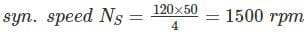Full load speed given as 1440 rpm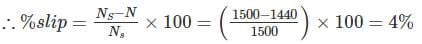Rotor voltage frequency = s × f = 0.04 × 50 = 5%
2 cycles/sec
⇒ 2 × 60 cycle/min = 120 cycles/min

Test: Electrical Machines- 5 - Question 2

### Under variable frequency operation of 3 phase Induction motor, air gap flux is kept constant by adjusting the motor voltage. For constant air gap flux, machine develops the same torque when the

Detailed Solution for Test: Electrical Machines- 5 - Question 2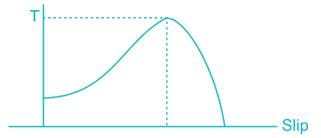In low slip region (stable region)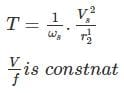Slip speed = SNs = Ns – Nr
Ns ∝ f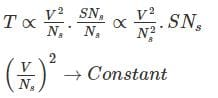Slip speed, SNs → Constant

*Answer can only contain numeric values
Test: Electrical Machines- 5 - Question 3

### The rotor winding of a 6 pole, 3 phase, slip-ring induction motor receive a 3-phase, 50 Hz supply produced an air gap mmf rotating in the clock wise direction with respect to the rotor the rotor itself is being rotated in the anticlockwise direction at 400 rpm. Frequency of the voltages induced in the stator phases is _______ Hz

Detailed Solution for Test: Electrical Machines- 5 - Question 3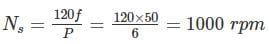Relating speed with respect to rotor = 1000
Speed with respect to stator = Nactual
Speed of rotor = Nr
1000 = Nactual – (-400)
Nactual = 600 R.P.M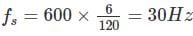Test: Electrical Machines- 5 - Question 4

A 6 – pole, 3 phase induction motor is drawing current from a 50Hz supply. If the rotor is running at a speed of 970 rpm, what is the speed of rotor magnetic field with respect to rotor?

Detailed Solution for Test: Electrical Machines- 5 - Question 4

Synchronous speed of stator field, NS = 120f/p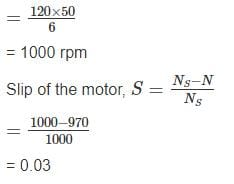Speed of rotor magnetic field relative to rotor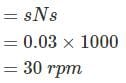*Answer can only contain numeric values
Test: Electrical Machines- 5 - Question 5

The ratio of starting to full load current for a 15 kW, 300 V and 3ϕ induction motor with DOL starter is _________. For 3 phase inductor motor has full load efficiency 0.90 the full load PF is 0.8 and short-circuit current is 30A at 100V.

Detailed Solution for Test: Electrical Machines- 5 - Question 5

Ist = 30 A
Starting current when it work at 300V
Ist = 30 × 3 = 90 A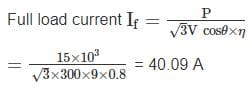∴ Ratio of starting current to full load current
= 90/40.09 = 2.244

Test: Electrical Machines- 5 - Question 6

A 208 V, 8 pole 60 Hz three phase star connected wound rotor induction motor has a rotor impedance of 0.02 + 0.08 Ω/phase and negligible stator impedance at standstill. Consider the following statements.
1. The breakdown slip at which maximum torque occurs is 0.25
2. The starting torque is 47% of the maximum torque
3. The maximum torque developed by the motor is 1364.4 Nm
Q. Which of the above statements is/are correct?

Detailed Solution for Test: Electrical Machines- 5 - Question 6

The breakdown slip is,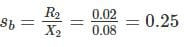The ratio of the torque developed at any slip s to the maximum torque is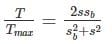For starting torque s = 1, so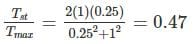Starting torque is 47% of maximum torque.
Synchronous speed,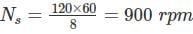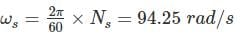Maximum power developed by the motor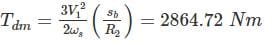*Answer can only contain numeric values
Test: Electrical Machines- 5 - Question 7

A 2 pole, 50 Hz induction motor supplies 15 kW to a load at a speed of 2950 rotation/min. How much power (In kW) will be supplied by the motor when the torque is doubled.

Detailed Solution for Test: Electrical Machines- 5 - Question 7

The synchronous speed of this motor is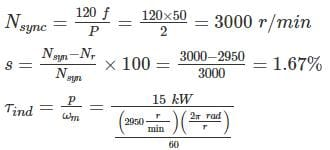= 48.6 N- m
In the low-slip region, the torque-speed curve is linear, and induced torque is directly proportional to slip. Therefore, if torque double, then the new slip will be 3.33 %. The operating speed of the motor is thus
Nr = (1 – .033) Nsyn = (1 -0.033) 3000 = 2900 r/min
∴ Power supplied = τind ω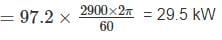*Answer can only contain numeric values
Test: Electrical Machines- 5 - Question 8

A 50 H.P, 4 pole, 50 Hz 3ϕ SRIM runs at 1440 RPM on full load with rotor current per phase of 30A. If it allows 1000 W for the mechanical loss then find the resistance per phase of the 3ϕ rotor winding.

Detailed Solution for Test: Electrical Machines- 5 - Question 8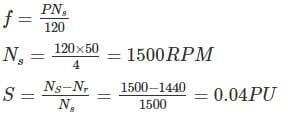We know that rotor copper loss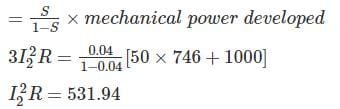900R = 531.94
R = 0.591Ω

Test: Electrical Machines- 5 - Question 9

A 3 ϕ squirrel cage induction motor has full load slip of 5% and starting current of 5 times the full load current. Neglect the stator and copper losses as well as the rotational losses. then the slip at which maximum torque occurs will be____.  Assume the stator impedance is negligible

Detailed Solution for Test: Electrical Machines- 5 - Question 9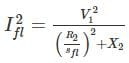Starting current,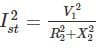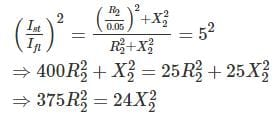R2/X2 = 24/375 = 0.25298

*Answer can only contain numeric values
Test: Electrical Machines- 5 - Question 10

The rotor resistance and standard reactance of a 3 phase induction motor are respectively 0.015 Ω and 0.09 Ω per phase. At normal voltage the full load slip is 3 %. Find the percentage reduction in stator voltage to develop full load torque at half full load speed.

Detailed Solution for Test: Electrical Machines- 5 - Question 10

Let Ns = 1000 r.p.m
Full load speed = [1 – 0.03] × 1000 = 970 rpm.
Let the normal voltage be V1 volts.
Speed in second case = 970/2 = 485 r. p.m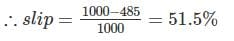We know that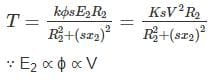Since Torque is the same in both case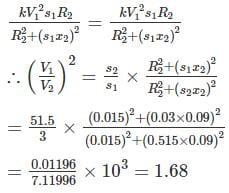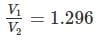Hence % reduction of stator voltage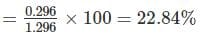## GATE Electrical Engineering (EE) 2023 Mock Test Series

22 docs|274 tests
Information about Test: Electrical Machines- 5 Page
In this test you can find the Exam questions for Test: Electrical Machines- 5 solved & explained in the simplest way possible. Besides giving Questions and answers for Test: Electrical Machines- 5, EduRev gives you an ample number of Online tests for practice

## GATE Electrical Engineering (EE) 2023 Mock Test Series

22 docs|274 tests(Scan QR code)